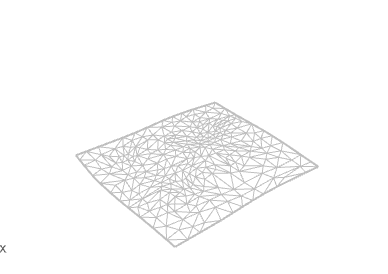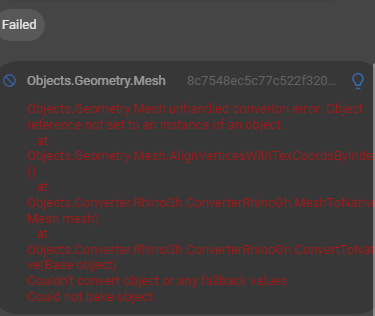# Rhino Mesh to Speckle Mesh

Hi everyone,

I’ve come across another problem.

``````res = requests.post(compute_url + "grasshopper", json=geo_payload)
for val in response_object:
paramName = val['ParamName']
innerTree = val['InnerTree']
for key, innerVals in innerTree.items():
for innerVal in innerVals:
if 'data' in innerVal:
mesh_geo = rh.CommonObject.Decode(data)
att = rh.ObjectAttributes()
att.LayerIndex = topography_layerIndex

vertices = mesh_geo.Vertices
faces = mesh_geo.Faces

vertex_list = [[vertices[i].X, vertices[i].Y, vertices[i].Z]
for i in range(len(vertices))]
vertex_list_flat = [coord for vertex in vertex_list for coord in vertex]
face_list = [(faces[i], faces[i], faces[i], faces[i]) for i in range(len(faces))]
flattened_faces = []
[flattened_faces.extend(list(face)) for face in face_list]

speckle_mesh = Mesh.create(vertices=vertex_list_flat, faces=flattened_faces)
``````

This is an extract from some code where I used a Rhino mesh to create a speckle mesh using the faces and vertices. I have double checked that the list of vertices and faces match the ones in Rhino by checking in Grasshopper, it seems to be the exact same. However, the speckle mesh is so distorted in the viewer.

Any idea why this is? Here is the commit of `speckle_mesh` I sent to a stream.

Commit | Speckle

ThanksThis is what the same mesh looks like in Rhino.

Assuming all of your mesh faces are triangles, try

``````face_list = [ (3, faces[i], faces[i], faces[i], faces[i]) for i in range(len(faces))]
``````

The signifier for vertex count per face is the `3` or it would be whatever the Rhino Mesh vertex count per face is. If you are producing meshes with NGONs then that 3 would become 4 or … whatever the count is

2 Likes

Hi Jonathan,

Works perfectly, thanks again!

One more question, when I try to receive the mesh in Rhino I get an error. Tried doing via GH, same problem.https://speckle.xyz/streams/16a7ca997a/commits/ded0a2d3cb

This is how I’m sending the data, just for reference again.

``````server_transport = ServerTransport(stream_id='16a7ca997a', client=client)
data =  speckle_mesh
speckle_geometry = speckle_objects.geometry.Mesh(data=data)
object_id = operations.send(
speckle_geometry, transports=[server_transport])
client.commit.create(stream_id='16a7ca997a', object_id=object_id)
``````

Currently, we are expecting the `textureCoordinates` list to be non `null` (not `none`)
In this case, I would suggest setting it to an empty list.

We probably could do a better job of communicating these requirements/validating objects better, especially on the SpecklePy side. We don’t do a great job of enforcing/documenting which properties need to be set and which ones don’t!

While we are at it, I would also suggest ensuring the `colors` list is initialized as an empty list also.
And setting `units` to one of these values.

3 Likes

Hi Jedd,

Thanks for the input, helped out a lot. I was also wondering how I could add the colors as well. I’ve further extended my code to include colors of the mesh into the speckle geometry. Something is definitely getting comitted but its not loading up.

Commit | Speckle

``````vertices = mesh_geo.Vertices
faces = mesh_geo.Faces
colors = mesh_geo.VertexColors

vertex_list = [[(vertices[i].X), (vertices[i].Y), vertices[i].Z]
for i in range(len(vertices))]
vertex_list_flat = [coord for vertex in vertex_list for coord in vertex]

face_list = [ (4, faces[i], faces[i], faces[i], faces[i])
for i in range(len(faces))]
flattened_faces = []
[flattened_faces.extend(list(face)) for face in face_list]

color_list = [(colors[i], colors[i], colors[i]) for i in range(len(colors))]
flattened_colors = []
[flattened_colors.extend(list(color)) for color in color_list]

speckle_mesh = Mesh.create(vertices=vertex_list_flat, faces=flattened_faces, texture_coordinates=[], colors=flattened_colors)

server_transport = ServerTransport(stream_id='eb641ee093', client=client)
data =  speckle_mesh
speckle_geometry = speckle_objects.geometry.Mesh(data=data)
object_id = operations.send(
speckle_geometry, transports=[server_transport])
client.commit.create(stream_id='eb641ee093', object_id=object_id)
``````

I notice your root commit object (`speckle_geometry`) is a nulled mesh.

I would suggest keeping your root commit object a `Base`.

Hi Jedd,

I actually did try with base first, got the same result so I changed it to a speckle mesh. I’ll give a try again and see again.

Thanks